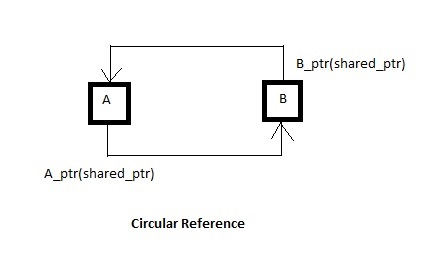## [LeetCode C++实现]406. Queue Reconstruction by Height

[[7,0], [4,4], [7,1], [5,0], ......

## exec家族execvp函数使用趣事

#include <stdio.h>

#include <stdlib.h>

#include <unistd.h>

#include <string.h>

#include <fcn......

## 地址空间随机化

common.h

#ifndef __common_h__

#define __common_h__

#include <sys/time.h>

#include <sys/stat.h>

#include <assert.h>

double GetTime() {

struct timeval t;

int rc = gettimeofday(&t, NULL);

assert(rc == 0);

return (double) t.tv_sec + (double) t.tv_usec......

## 日常开发笔记总结(五）

#include <stdio.h>

//获取下标为n的元素地址

#define ELEM_PTR(base,n,esize) ((void *)(((char *)base + n*esize)))

int main()

{

int arr[] = {1,2,3,4,5,6,7,8,9,10};

printf("address of fifth elem = %p.\n",ELEM_PTR(arr,5,4......

## 日常开发笔记总结(四）a.h

#ifndef A_H

#define A_H

class B; //forward declaration

cl......

## [LeetCode C++实现]46.Permutations

46.Permutations

next_permutation

class Solution {

public:

vector<vector<int> > permute(vector<int> &num) {

vector<vector<int> > ans;

sort(num.begin(), num......

## KMP算法简介与应用

class Solution {

public:

int strStr(string haystack, string needle) {

int hsize = haystack.size();

int nsize = needle.size();

for......

## c++filt用法介绍

typedef char * pstring;

const pstring cstr = 0; //cstr是指向char的常量指针

const pstring *ps; //ps是一个指针，它的对象是指向char的常量指针

typedef char *......

## C++语言导学

[root workspace]#g++ --version | head -1

g++......